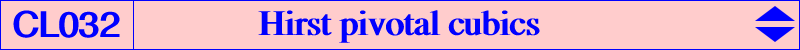Let U = u : v : w be the perspector of a circum-conic (C), with center Q = G-Ceva conjugate of U = u(v + w - u) : : . For any point P on (C), the pivotal cubic with pivot P, invariant in the isoconjugation which swaps P and Q is denoted by pK(P x Q, P). This cubic is naturally invariant under this isoconjugation and under P-Ceva conjugation but also under a third quadratic transformation : the Hirst inversion with pole Q and conic (C). Let us remind that this Hirst inversion maps any point M (distinct of Q) to the intersection M' of the line MQ and the polar line of M in (C). From this we can see that this Hirst inversion coincide with P-Ceva conjugation for any point M on the cubic since Q is the isopivot (or secondary pivot). Indeed, pK(P x Q, P) is the locus of M such that M, P/M and Q are collinear, where P/M is the P-Ceva conjugate of M. This Hirst inversion generalizes the inversion in the circumcircle which is obtained when U = K, the Lemoine point. For a given point U, all these pK(P x Q, P) form a pencil of pK since they all pass through A, B, C, Q, the points at infinity of (C) and since their tangents at A, B, C pass through Q. From this we see that these cubics have always two common asymptotic directions which are real, imaginary, identical if and only if U lies outside, inside, on the Steiner inscribed ellipse respectively. The cubic of the pencil which contains P on (C) must go through P-Ceva Q (the third point at infinity), its isoconjugate, the isoconjugates of the points at infinity of (C). Furthermore, • (C) is a circle if and only if U = K in which case pK(P x Q, P) is a circular inversible cubic such as K112, K113, K114 (see Special Isocubics §4.4). • (C) is a rectangular hyperbola if and only if U lies on the orthic axis in which case the cubic has two perpendicular asymptotes. See K237 and K238 for example. • When U = G hence Q = G, (C) is the Steiner ellipse and P x Q = P. We obtain cubics of the class CL007.